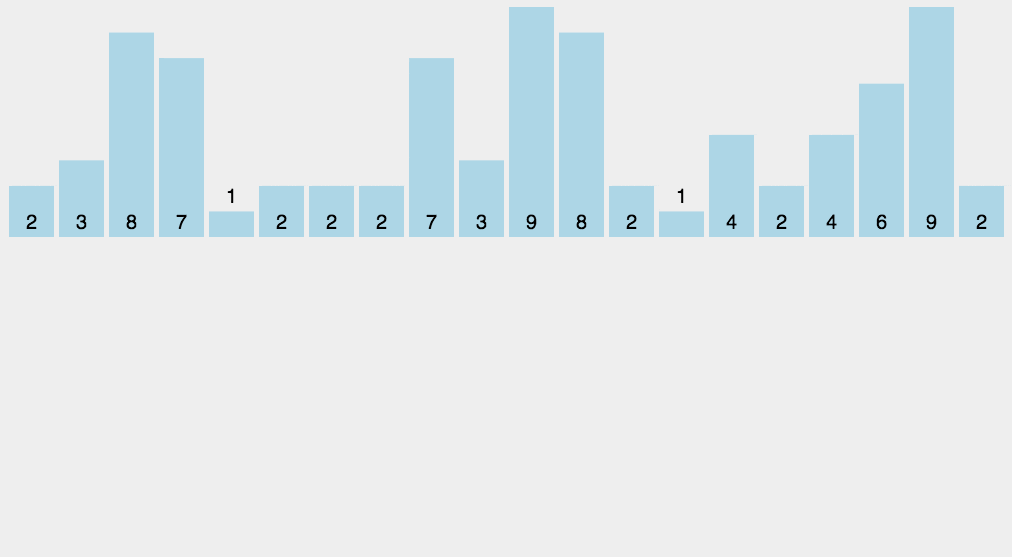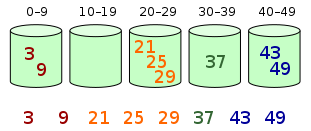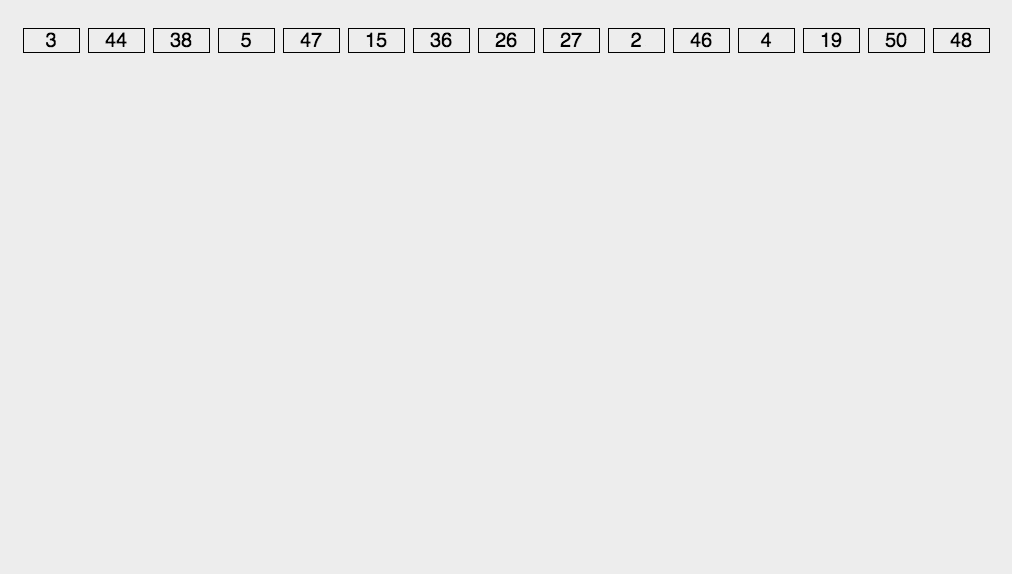# # 经典排序算法

• 计数排序：每个桶只存储单一键值
• 桶排序：每个桶存储一定范围的数值
• 基数排序：根据键值的每位数字来分配桶

## # 8. 计数排序

• 一个县百万人的年龄排序
• 高考几百万考生的成绩排序

### # 8.1. 算法步骤• 找出待排序的数组中最大和最小的元素
• 统计数组中每个值为 value 的元素出现的次数，存入数组 bucket 的第 value 项
• 对所有的计数累加（从 bucket 中的第一个元素开始，每一项和前一项相加）
• 反向填充目标数组：将每个元素 value 放在新数组的第 bucket(value) 项，每放一个元素就将 bucket(value) 减去 1

### # 8.2. 基础版本

``````/**
* 计数排序基础版本，不稳定，不支持负数
* 使用最大值+1计算需要几个bucket
*
* 只用最大值计算这样会导致bucket过大浪费
*
* @param array
*/
private static void countingSort1(int[] array) {
print(array);

// 找到最大值计算需要几个bucket
int maxValue = 0;
for (int value : array) {
if (maxValue < value) {
maxValue = value;
}
}
// 创建bucket
int bucketLen = maxValue + 1;
int[] bucket = new int[bucketLen];
// 遍历数组，将值放入bucket中
for (int value : array) {
bucket[value]++;
}
// 输出bucket的值
for (int i = 0, pos = 0; i < bucketLen; i++) {
while (bucket[i] > 0) {
array[pos] = i;
pos++;
bucket[i]--;
}
}

System.out.println("bucketLen " + bucketLen);
print(array);
}
``````

``````public static void main(String[] args) {
int[] array = {5, 12, 10, 9, 6, 9, 7, 9, 9, 8, 5, 7, 8, 5, 6, 6, 6, 5, 7, 9, 8, 5, 15};
System.out.println("---------- countingSort1 ----------");
countingSort1(Arrays.copyOf(array, array.length));
}
``````
``````---------- countingSort1 ----------
5 12 10 9 6 9 7 9 9 8 5 7 8 5 6 6 6 5 7 9 8 5 15
bucketLen 16
5 5 5 5 5 6 6 6 6 7 7 7 8 8 8 9 9 9 9 9 10 12 15
``````

### # 8.3. 优化版本

``````/**
* 计数排序优化版本，不稳定，支持负数
* 使用最大值和最小值计算极值差+1个bucket
*
* 可以看到bucket数量变小了
* 存数时减去最小值，支持负数
*
* @param array
*/
private static void countingSort2(int[] array) {
print(array);

// 找到最大值和最小值计算需要几个bucket
int maxValue = array, minValue = array;
for (int value : array) {
if (maxValue < value) {
maxValue = value;
} else if (minValue > value) {
minValue = value;
}
}
// 创建bucket
int bucketLen = maxValue - minValue + 1;
int[] bucket = new int[bucketLen];
// 遍历数组，将值放入bucket中
for (int value : array) {
// 优化减小了bucket的大小
int index = value - minValue;
bucket[index]++;
// bucket[value - minValue]++;
}
// print(bucket);
// 输出bucket的值，按存取的方式取出元素
for (int i = 0, pos = 0; i < bucket.length; i++) {
// 根据bucket数组中存的次数去取排列好的元素
while (bucket[i] > 0) {
array[pos] = minValue + i;
pos++;
bucket[i]--;
}
}
}
``````

``````public static void main(String[] args) {
int[] array2 = {5, 12, 10, 9, 6, 9, 7, 9, -9, -8, 5, 7, 8, 5, 6, 6, -6, -5, 7, 9, 8, 5, 15};
System.out.println("---------- countingSort2 ----------");
countingSort2(Arrays.copyOf(array2, array2.length));
}
``````
``````---------- countingSort2 ----------
5 12 10 9 6 9 7 9 -9 -8 5 7 8 5 6 6 -6 -5 7 9 8 5 15
bucketLen 25
-9 -8 -6 -5 5 5 5 5 6 6 6 7 7 7 8 8 9 9 9 9 10 12 15
``````

### # 8.4. 优化版本v2

``````/**
* 计数排序优化版本v2，利用累加数组做到算法稳定，不支持负数
* 使用最大值计算需要几个bucket
*
* @param array
*/
private static void countingSort3(int[] array) {
print(array);

// 找到最大值计算需要几个bucket
int maxValue = 0;
for (int value : array) {
if (maxValue < value) {
maxValue = value;
}
}
// 创建bucket
int bucketLen = maxValue + 1;
int[] bucket = new int[bucketLen];
// 遍历数组，将值放入bucket中
for (int value : array) {
bucket[value]++;
}

print(bucket);

int[] tempArray = new int[array.length];
// 利用累加数组计算出元素下标
for (int i = 1; i < bucket.length; i++) {
bucket[i] = bucket[i] + bucket[i - 1];
}

print(bucket);

// 利用累加数组的方式取出元素，从最后一位开始
for (int i = array.length - 1; i >= 0; i--) {
// 取出原数组每一位的值
int value = array[i];
// 将其-1后得到的就是它的在bucket中的下标
bucket[value]--;
// 根据bucket加下标得到该元素最终在有序数组中的下标
int pos = bucket[value];
// 赋值到新数组对应的下标位置
tempArray[pos] = array[i];
}

System.out.println("bucketLen " + bucketLen);
print(tempArray);
}
``````

``````public static void main(String[] args) {
int[] array = {5, 12, 10, 9, 6, 9, 7, 9, 9, 8, 5, 7, 8, 5, 6, 6, 6, 5, 7, 9, 8, 5, 15};
System.out.println("---------- countingSort3 ----------");
countingSort3(Arrays.copyOf(array, array.length));
}
``````
``````---------- countingSort3 ----------
5 12 10 9 6 9 7 9 9 8 5 7 8 5 6 6 6 5 7 9 8 5 15
0 0 0 0 0 5 4 3 3 5 1 0 1 0 0 1
0 0 0 0 0 5 9 12 15 20 21 21 22 22 22 23
bucketLen 16
5 5 5 5 5 6 6 6 6 7 7 7 8 8 8 9 9 9 9 9 10 12 15
``````

### # 8.5. 优化版本v3

``````/**
* 计数排序优化版本v3，利用累加数组做到算法稳定，支持负数
* 使用最大值和最小值计算极值差+1个bucket
* 存数时减去最小值，支持负数
*
* @param array
*/
private static void countingSort4(int[] array) {
print(array);

// 找到最大值和最小值计算需要几个bucket
int maxValue = array, minValue = array;
for (int value : array) {
if (maxValue < value) {
maxValue = value;
} else if (minValue > value) {
minValue = value;
}
}
// 创建bucket
int bucketLen = maxValue - minValue + 1;
int[] bucket = new int[bucketLen];
// 遍历数组，将值放入bucket中
for (int value : array) {
// 优化减小了bucket的大小
int index = value - minValue;
bucket[index]++;
// bucket[value - minValue]++;
}

// print(bucket);

int[] tempArray = new int[array.length];
// 利用累加数组计算出元素下标
for (int i = 1; i < bucket.length; i++) {
bucket[i] = bucket[i] + bucket[i - 1];
}

// print(bucket);

// 利用累加数组的方式取出元素，从最后一位开始
for (int i = array.length - 1; i >= 0; i--) {
// 取出原数组每一位的值
int value = array[i] - minValue;
// 将其-1后得到的就是它的在bucket中的下标
bucket[value]--;
// 根据bucket加下标得到该元素最终在有序数组中的下标
int pos = bucket[value];
// 赋值到新数组对应的下标位置
tempArray[pos] = array[i];
}

System.out.println("bucketLen " + bucketLen);
// print(array);
print(tempArray);
}
``````

``````public static void main(String[] args) {
int[] array2 = {5, 12, 10, 9, 6, 9, 7, 9, -9, -8, 5, 7, 8, 5, 6, 6, -6, -5, 7, 9, 8, 5, 15};
System.out.println("---------- countingSort4 ----------");
countingSort4(Arrays.copyOf(array2, array2.length));
}
``````
``````---------- countingSort4 ----------
5 12 10 9 6 9 7 9 -9 -8 5 7 8 5 6 6 -6 -5 7 9 8 5 15
bucketLen 25
-9 -8 -6 -5 5 5 5 5 6 6 6 7 7 7 8 8 9 9 9 9 10 12 15
``````

### # 8.6. 算法总结

• 当待排序序列的最大值和最小值差值特别大时，不适合使用计数排序算法
• 当待排序序列的值不是整数时，不适合使用计数排序算法

• 描述
• 统计小于等于该元素值的元素个数 i，于是该元素就放在目标数组的索引 i 位(i > 0)
• 稳定性
• 稳定的
• 时间复杂度
• 最好是 O(n + k)
• 最差为 O(n + k)
• 所以平均复杂度为 O(n + k)
• 空间复杂度
• 占用额外空间，为 O(k)

## # 9. 桶排序

• 在额外空间充足的情况下，尽量增大桶的数量
• 使用的映射函数能够将输入的 N 个数据均匀的分配到 K 个桶中

• 当输入的数据可以均匀的分配到每一个桶中速度最快
• 当输入的数据被分配到了同一个桶中速度最慢

### # 9.1. 算法步骤• 设置一个定量的数组当作空桶
• 遍历输入数据，并且把数据一个一个放到对应的桶里去
• 对每个不是空的桶进行排序
• 从不是空的桶里把排好序的数据拼接起来

### # 9.2. 基础版本

``````/**
* 桶排序基础版本，使用数组实现，指定bucketSize为5
* 存数时减去最小值，支持负数
*
* @param array
*/
private static void bucketSort1(int[] array) {
print(array);

int bucketSize = 5;
int minValue = array;
int maxValue = array;
for (int value : array) {
if (value < minValue) {
minValue = value;
} else if (value > maxValue) {
maxValue = value;
}
}

int bucketCount = (int) Math.floor((maxValue - minValue) / bucketSize) + 1;
int[][] buckets = new int[bucketCount];

// 利用映射函数将数据分配到各个桶中
for (int i = 0; i < array.length; i++) {
int index = (int) Math.floor((array[i] - minValue) / bucketSize);
buckets[index] = arrAppend(buckets[index], array[i]);
}

int pos = 0;
for (int[] bucket : buckets) {
if (bucket.length <= 0) {
continue;
}
// 对每个桶进行排序，这里使用了插入排序
bucket = insertSort(bucket);
for (int value : bucket) {
array[pos] = value;
pos++;
}
}

print(array);
}

/**
* 自动扩容，并保存数据
*
* @param array
* @param value
*/
private static int[] arrAppend(int[] array, int value) {
array = Arrays.copyOf(array, array.length + 1);
array[array.length - 1] = value;
return array;
}

/**
* 插入排序
*
* @param array
* @return
*/
private static int[] insertSort(int[] array) {
for (int i = 1; i < array.length; i++) {
int current = array[i];
int j = i;
while (j > 0 && (current < array[j - 1])) {
array[j] = array[j - 1];
j--;
}
if (j != i) {
array[j] = current;
}
}
return array;
}
``````

``````public static void main(String[] args) {
int[] array = {5, 12, 10, 9, 6, 9, 7, 9, -9, -8, 5, 7, 8, 5, 6, 6, -6, -5, 7, 9, 8, 5, 15};
System.out.println("---------- bucketSort1 ----------");
bucketSort1(Arrays.copyOf(array, array.length));
}
``````
``````---------- bucketSort1 ----------
5 12 10 9 6 9 7 9 -9 -8 5 7 8 5 6 6 -6 -5 7 9 8 5 15
-9 -8 -6 -5 5 5 5 5 6 6 6 7 7 7 8 8 9 9 9 9 10 12 15
``````

### # 9.3. ArrayList实现

``````/**
* 桶排序使用ArrayList实现，指定bucketSize为5
* ArrayList支持负数存取
*
* @param array
*/
private static void bucketSort2(int[] array) {
print(array);

int bucketSize = 5;
List[] buckets = new ArrayList[bucketSize];
// 初始化
for (int i = 0; i < buckets.length; i++) {
buckets[i] = new ArrayList<Integer>();
}
// 将待排序序列放入对应桶中
for (int i = 0; i < array.length; i++) {
// 计算对应的桶号
int index = array[i] / 10;
}
// 排序输出
for (int k = 0, pos = 0; k < buckets.length; k++) {
// 每个桶内进行排序
buckets[k].sort(null);
for (int j = 0; j < buckets[k].size(); j++) {
array[pos] = (int) buckets[k].get(j);
pos++;
}
}

print(array);
}
``````

``````public static void main(String[] args) {
int[] array = {5, 12, 10, 9, 6, 9, 7, 9, -9, -8, 5, 7, 8, 5, 6, 6, -6, -5, 7, 9, 8, 5, 15};
System.out.println("---------- bucketSort2 ----------");
bucketSort2(Arrays.copyOf(array, array.length));
}
``````
``````---------- bucketSort2 ----------
5 12 10 9 6 9 7 9 -9 -8 5 7 8 5 6 6 -6 -5 7 9 8 5 15
-9 -8 -6 -5 5 5 5 5 6 6 6 7 7 7 8 8 9 9 9 9 10 12 15
``````

### # 9.4. 算法总结

• 描述
• 将值为 i 的元素放入 i 号桶，将桶内元素排序，最后依次把桶里的元素倒出来
• 稳定性
• 一般是稳定的，当桶内排序使用不稳定算法，才会导致不稳定
• 时间复杂度
• 最好是 O(n + k)
• 最差为 O(n^2)
• 所以平均复杂度为 O(n + k)
• 空间复杂度
• 占用额外空间，为 O(n + k)

## # 10. 基数排序

• 最高位优先法(Most Significant Digit first)，简称 MSD 法
• 最低位优先法(Least Significant Digit first)，简称 LSD 法

### # 10.1. 算法步骤

(LSD)动图演示(LSD)算法步骤

• 取得数组中的最大数，并取得位数
• 位数较短的通过补 0 达到长度一致
• 重复每位数进行排序后，再将各组连接起来，便得到一个有序序列

### # 10.2. 基础版本

``````/**
* 基数排序基础版本，不支持负数
*
* @param array
*/
private static void radixSort1(int[] array) {
print(array);

int[] tempArray = new int[array.length];
int[] counter = new int;

// 使用最大值计算位数
int maxValue = array;
for (int value : array) {
if (maxValue < value) {
maxValue = value;
}
}
// 获取位数
int maxDigit = getNumLength(maxValue);
for (int i = 0; i < maxDigit; i++) {
int division = (int) Math.pow(10, i);
// System.out.println(division);

for (int j = 0; j < array.length; j++) {
int num = array[j] / division % 10;
counter[num]++;
}

// 累加数组保证稳定
for (int m = 1; m < counter.length; m++) {
counter[m] = counter[m] + counter[m - 1];
}

// 利用累加数组的方式取出元素，从最后一位开始
for (int n = array.length - 1; n >= 0; n--) {
// 取出所在counter下标
int num = array[n] / division % 10;
// 将其-1后得到的就是它的在counter中的下标
counter[num]--;
// 根据counter加下标得到该元素最终在有序数组中的下标
int pos = counter[num];
// 赋值到新数组对应的下标位置
tempArray[pos] = array[n];
}

// 将tempArray复制到array
// System.arraycopy(tempArray, 0, array, 0, array.length);
array = Arrays.copyOf(tempArray, tempArray.length);
// 将counter数组值全部填充置为0，重新开始下一轮
Arrays.fill(counter, 0);

print(array);
}

// print(array);
}

/**
* 获取数字位数
*
* @param num
* @return
*/
private static int getNumLength(long num) {
if (num == 0) {
return 1;
}
int length = 0;
for (long temp = num; temp != 0; temp /= 10) {
length++;
}
return length;
}
``````

• 第 1 趟按个位数，将个位数的先排序好
• 第 2 趟按十位数，再将十位数的排序好
• 然后百位数，位数较短的缺失的位数默认为 0，原来的个位和十位数就按顺序到前面去了
• 千位数也是一样，最终排序成功
``````public static void main(String[] args) {
int[] array = {5, 122, 102, 95, 63, 70, 88, 73, 88, 81, 555, 666, 729, 19, 28, 45, 1115};
}
``````
``````---------- radixSort1 ----------
5 122 102 95 63 70 88 73 88 81 555 666 729 19 28 45 1115
70 81 122 102 63 73 5 95 555 45 1115 666 88 88 28 729 19
102 5 1115 19 122 28 729 45 555 63 666 70 73 81 88 88 95
5 19 28 45 63 70 73 81 88 88 95 102 1115 122 555 666 729
5 19 28 45 63 70 73 81 88 88 95 102 122 555 666 729 1115
``````

### # 10.3. 优化版本

``````/**
* 基数排序优化版本，支持负数
*
* 考虑负数的情况，扩展一倍队列数
* 其中[0-9]对应负数，[10-19]对应正数(bucket + 10)
*
* @param array
*/
private static void radixSort2(int[] array) {
print(array);

int[] tempArray = new int[array.length];
// 考虑负数的情况，扩展一倍队列数，其中[0-9]对应负数，[10-19]对应正数(bucket + 10)
int[] counter = new int;

// 使用最大值计算位数
int maxValue = array;
for (int value : array) {
if (maxValue < value) {
maxValue = value;
}
}
// 获取位数
int maxDigit = getNumLength(maxValue);
for (int i = 0; i < maxDigit; i++) {
int division = (int) Math.pow(10, i);
// System.out.println(division);

for (int j = 0; j < array.length; j++) {
int num = array[j] / division % 10;
// 值大于等于0，存放在10-19的正数区域
// 其中 [0-9]对应负数，[10-19]对应正数 (bucket + 10)
num = num + 10;
counter[num]++;
}

// 累加数组保证稳定
for (int m = 1; m < counter.length; m++) {
counter[m] = counter[m] + counter[m - 1];
}

// 利用累加数组的方式取出元素，从最后一位开始
for (int n = array.length - 1; n >= 0; n--) {
// 取出所在counter下标
int num = array[n] / division % 10;
// 值大于等于0，存放在10-19的正数区域
// 其中 [0-9]对应负数，[10-19]对应正数 (bucket + 10)
num = num + 10;
// 将其-1后得到的就是它的在counter中的下标
counter[num]--;
// 根据counter加下标得到该元素最终在有序数组中的下标
int pos = counter[num];
// 赋值到新数组对应的下标位置
tempArray[pos] = array[n];
}

// 将tempArray复制到array
// System.arraycopy(tempArray, 0, array, 0, array.length);
array = Arrays.copyOf(tempArray, tempArray.length);
// 将counter数组值全部填充置为0，重新开始下一轮
Arrays.fill(counter, 0);

print(array);
}

// print(array);
}
``````

• 第 1 趟按个位数，将个位数的先排序好
• 第 2 趟按十位数，再将十位数的排序好
• 然后百位数，位数较短的缺失的位数默认为 0，原来的个位和十位数就按顺序到前面去了
• 千位数也是一样，最终排序成功
``````public static void main(String[] args) {
int[] array2 = {5, 122, 102, 95, 63, -7, 88, 73, 88, 81, -555, -666, 729, -19, 28, 45, 1115};
}
``````
``````---------- radixSort2 ----------
5 122 102 95 63 -7 88 73 88 81 -555 -666 729 -19 28 45 1115
-19 -7 -666 -555 81 122 102 63 73 5 95 45 1115 88 88 28 729
-666 -555 -19 -7 102 5 1115 122 28 729 45 63 73 81 88 88 95
-666 -555 -19 -7 5 28 45 63 73 81 88 88 95 102 1115 122 729
-666 -555 -19 -7 5 28 45 63 73 81 88 88 95 102 122 729 1115
``````

### # 10.3. 优化版本v2

``````/**
* 基数排序优化版本v2，使用二维数组实现，支持负数
*
* @param array
*/
private static void radixSort3(int[] array) {
print(array);

// 使用最大值计算位数
int maxValue = array;
for (int value : array) {
if (maxValue < value) {
maxValue = value;
}
}
// 获取位数
int maxDigit = getNumLength(maxValue);
int mod = 10;
int dev = 1;

for (int i = 0; i < maxDigit; i++, dev = dev * 10, mod = mod * 10) {
// 考虑负数的情况，扩展一倍队列数，其中[0-9]对应负数，[10-19]对应正数(bucket + 10)
int[][] counter = new int[mod * 2];

// 放入桶
for (int j = 0; j < array.length; j++) {
int bucket = ((array[j] % mod) / dev) + mod;
counter[bucket] = arrayAppend(counter[bucket], array[j]);
}

// 稳定的，使用二维数组存放了值
int pos = 0;
for (int[] bucket : counter) {
for (int value : bucket) {
array[pos++] = value;
}
}

print(array);
}

// print(array);
}

/**
* 自动扩容，并保存数据
*
* @param arr
* @param value
*/
private static int[] arrayAppend(int[] arr, int value) {
arr = Arrays.copyOf(arr, arr.length + 1);
arr[arr.length - 1] = value;
return arr;
}
``````

• 第 1 趟按个位数，将个位数的先排序好
• 第 2 趟按十位数，再将十位数的排序好
• 然后百位数，位数较短的缺失的位数默认为 0，原来的个位和十位数就按顺序到前面去了
• 千位数也是一样，最终排序成功
``````public static void main(String[] args) {
int[] array2 = {5, 122, 102, 95, 63, -7, 88, 73, 88, 81, -555, -666, 729, -19, 28, 45, 1115};
}
``````
``````---------- radixSort3 ----------
5 122 102 95 63 -7 88 73 88 81 -555 -666 729 -19 28 45 1115
-19 -7 -666 -555 81 122 102 63 73 5 95 45 1115 88 88 28 729
-666 -555 -19 -7 102 5 1115 122 28 729 45 63 73 81 88 88 95
-666 -555 -19 -7 5 28 45 63 73 81 88 88 95 102 1115 122 729
-666 -555 -19 -7 5 28 45 63 73 81 88 88 95 102 122 729 1115
``````

### # 10.5. 算法总结

• 描述
• 一种多关键字的排序算法
• 稳定性
• 稳定的
• 时间复杂度
• 最好是 O(n * k)
• 最差为 O(n * k)
• 所以平均复杂度为 O(n * k)
• 空间复杂度
• 占用额外空间，为 O(n + k)